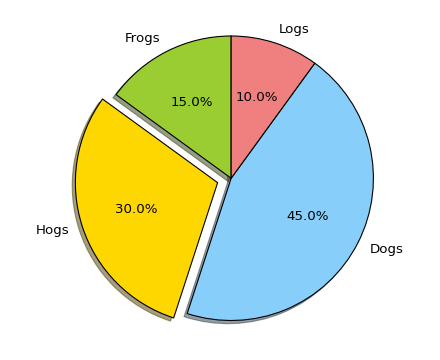### We're updating the default styles for Matplotlib 2.0

Learn what to expect in the new updates#### Previous topic

pie_and_polar_charts Examples

#### Next topic

pie_and_polar_charts example code: polar_bar_demo.py

# pie_and_polar_charts example code: pie_demo_features.py¶```"""
Demo of a basic pie chart plus a few additional features.

In addition to the basic pie chart, this demo shows a few optional features:

* slice labels
* auto-labeling the percentage
* offsetting a slice with "explode"
* drop-shadow
* custom start angle

Note about the custom start angle:

The default ``startangle`` is 0, which would start the "Frogs" slice on the
positive x-axis. This example sets ``startangle = 90`` such that everything is
rotated counter-clockwise by 90 degrees, and the frog slice starts on the
positive y-axis.
"""
import matplotlib.pyplot as plt

# The slices will be ordered and plotted counter-clockwise.
labels = 'Frogs', 'Hogs', 'Dogs', 'Logs'
sizes = [15, 30, 45, 10]
colors = ['yellowgreen', 'gold', 'lightskyblue', 'lightcoral']
explode = (0, 0.1, 0, 0) # only "explode" the 2nd slice (i.e. 'Hogs')

plt.pie(sizes, explode=explode, labels=labels, colors=colors,
autopct='%1.1f%%', shadow=True, startangle=90)
# Set aspect ratio to be equal so that pie is drawn as a circle.
plt.axis('equal')

plt.show()
```

Keywords: python, matplotlib, pylab, example, codex (see Search examples)